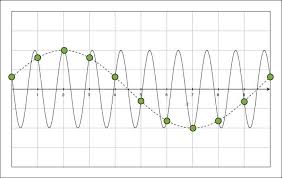Basic Concepts

05.05.2020

Signals

Analog Signal

It is a signal that has a continuous nature rather than a pulsed or discrete one.

Analog Signal varies continually. Because of a continuous function, It consists of an infinite number of values.

Digital Signal

Only takes discrete values. Digital Signal takes only discrete & computer storable values.

Analog and digital signals are used to transmit information, usually through electric signals.

In both these technologies, the information, such as any audio or video, is transformed into electric signals.

The difference between analog and digital technologies is that in analog technology, information is translated into electric pulses of varying amplitude. In digital technology, the translation of information is into binary format (zero or one) where each bit is representative of two distinct amplitudes.

We need to convert analog signals to digital numbers

• OTHERWISE

- We cannot display them on digital screens.

- We cannot store them on servers/computers

- We cannot apply digital filters on them (to remove noise etc.)

- We cannot make automatic calculations on them

- WE CANNOT EVALUATE AND USE EASILY.

Sampling of 1D Signals

Sampling Period (T): the time between 2 consecutive samples.

Sampling rate/frequency (fs=1/T): acquired samples in one second.

Aliasing

A type of distortion that occurs when digitally recording high frequencies with a low sample rate.

Circles: Sampled signal points

Red: Analog signal,

Blue: Reconstituted (from sampled points) analog signal NOTE THAT aliasing occurs (Volt vs. second).

Sampling of 2D Signals

There are TWO sampling rates (or periods) for 2 dimensions.

SAMPLING OF 2D SIGNALS => X-Ray film 2D matrix of numbers.

Sampling of  3D Signals

There are THREE sampling rates/periods for 3 dimensions.

Interpolation, Decimation and Downsampling of Digitial Signals

Interpolation is a method of constructing new data points within the range of a discrete set of known data points.

Downsampling (or "subsampling") is the process of reducing the sampling rate of a signal.

Decimation is a technique for reducing the number of samples in a digital signal. It is a two step process:

- 1. Low-pass anti-aliasing filter, then

- 2. Downsampling

Interpolation in 1D

• There are many methods for interpolation

• In the following figure, empty parts of the signal in A, were filled with different (B, C, D) interpolation methods

Interpolation increases the size of the signal vector/matrix

X: original signal

Y: interpolated signal

Length of Y > Length of X

Interpolation in 2D

There are many methods for interpolation in 2D also

Example: In color doppler imaging (CDI), every color corresponds to a velocity

Many pixels are INTERPOLATED on the image.

Question marks are filled w.r.t. their neighbors

Sometimes, errors can be seen due to interpolation

More Definitions

Amplitude: the greatness of magnitude

Resolution: capability of making distinguishable the neighbor parts of an object.

There are many definitions w.r.t. topic (CT, MRI, USG videos, images, etc.).

- The term resolution is often used as a pixel count in digital imaging etc.

DIGITAL SIGNAL COMPRESSION

Definitions

- 1 Byte=8-bit

- 1 KByte=103 byte,

- 1 MByte=106 byte,

- 1 GByte=109 byte,

- 1 TByte=1012 byte

An ECG signal: 16-bit x 1000 sample/sec - Bit-rate (Byte/s)=16,000 bit/s=2 KByte/s

- Traditional ECG has 10 channels, so Bit-rate= 8x20 KByte/s

• a Rx image has 4000x4000 pixel and each pixel is represented with 16 bit gray value => 4000x4000x2 bytes =32 MBytes

• A medical video has 24 bits RGB pixels +1000x600 image frames + 30 fps video speed (3x1000x600@30 fps).

- Bit-rate =8x3.1000.600.30 Byte/s=54.106 Byte/s ≈ 4.108 Bit/s

• Compressing medical signals for video and Rx is a requirement

Filters

* Filters are devices/programs so that stop or pass some parts of the frequency spectrum.

• Low pass filter: passes only low frequencies

• High pass filter: passes only high frequencies

• Bandpass filter: passes only a band of spectrum

• Bandstop filter: stops only a band of spectrum

Filter Types

(Filter Amplitude vs. Frequency graphs of filters)

Low Pass (LP) filter, the cut-off frequency is 0.5 Hz

Band Stop (BS) filter, cut-off frequencies are wL and wH

Band Pass (BP) filter, cut-off frequencies are f1 and f2

High Pass (HP) filter, the cut-off frequency is 10 Hz